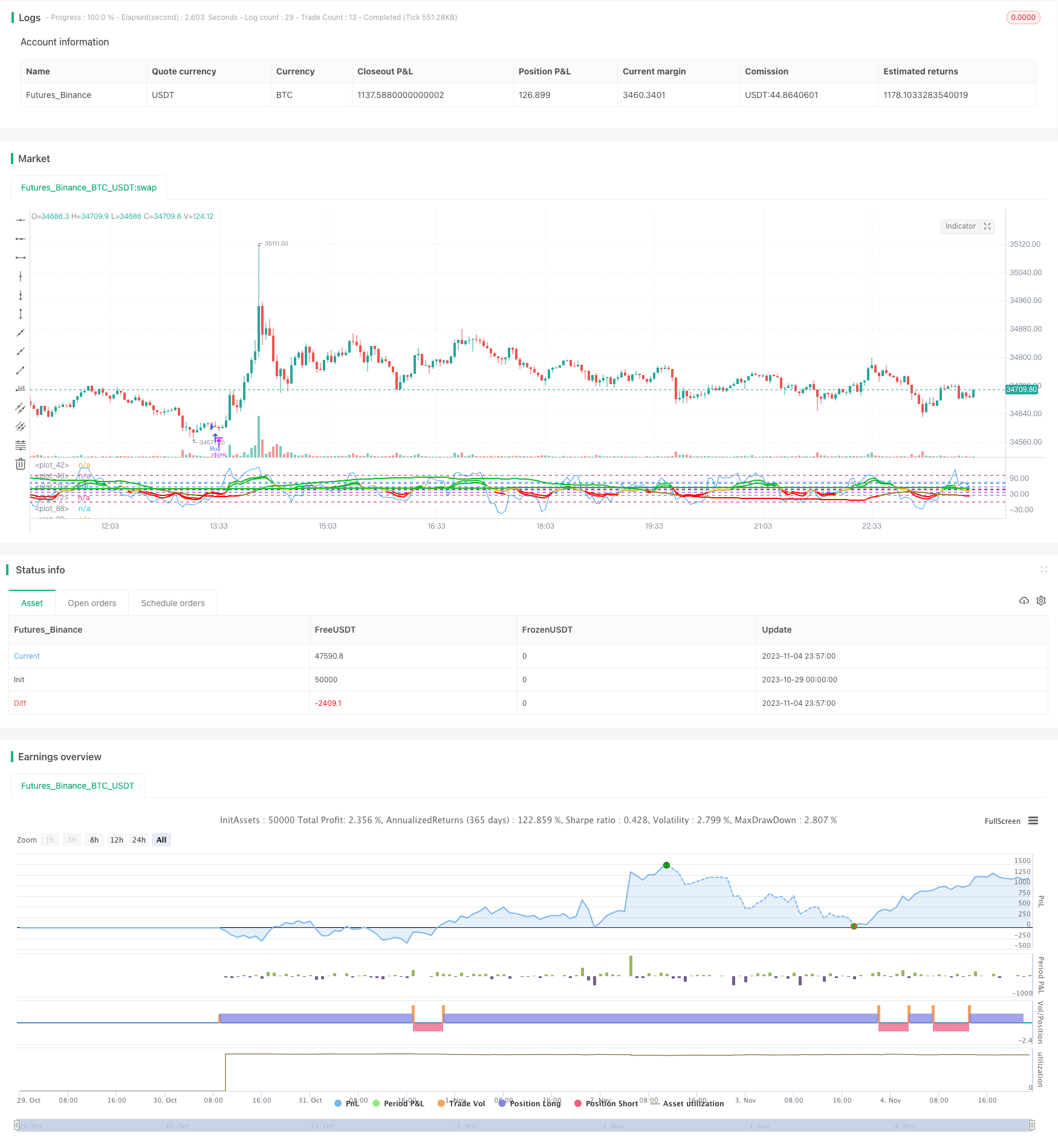# Super Momentum Strategy

Author: ChaoZhang, Date: 2023-11-06 09:24:02
Tags:## Overview

The Super Momentum strategy combines multiple momentum indicators. It buys when multiple momentum indicators are bullish concurrently, and sells when they are bearish concurrently. By integrating multiple momentum indicators, it aims to capture price trends more accurately and avoid false signals from individual indicators.

## Strategy Logic

The strategy uses 4 RMI indicators by Everget and 1 Chande Momentum Oscillator. RMI measures price momentum to gauge bullish and bearish strength. Chande MO calculates price change to identify overbought and oversold conditions.

It goes long when RMI5 crosses above its buy line, RMI4 crosses below its buy line, RMI3 crosses below its buy line, RMI2 crosses below its buy line, RMI1 crosses below its buy line, and Chande MO crosses above its buy line.

It goes short when RMI5 crosses below its sell line, RMI4 crosses above its sell line, RMI3 crosses above its sell line, RMI2 crosses above its sell line, RMI1 crosses above its sell line, and Chande MO crosses below its sell line.

RMI5 is set opposite to other RMI to better identify trends for pyramid trading.

• Combining multiple indicators improves trend accuracy and avoids false signals

• Indicators across timeframes catch larger trends

• Reverse RMI aids in trend identification and pyramiding

## Risk Analysis

• Complex parameters with multiple indicators need thorough optimization

• Concurrent indicator moves may generate false signals

• Lower trade frequency with multiple filters

• Parameters may not suit different products and market regimes

## Optimization Directions

• Test and optimize parameters for strategy robustness

• Add/remove indicators to evaluate signal quality impact

• Introduce filters to avoid false signals in certain markets

• Consider adding stop loss for risk control

## Conclusion

This strategy improves trend judgment by integrating momentum indicators. But parameter optimization is crucial due to complexity. If well-tuned, it can generate quality signals and has an edge in trend following. But traders should watch for risks, find optimal parameters, and incorporate risk controls for steady trading.

```/*backtest
start: 2023-10-29 00:00:00
end: 2023-11-05 00:00:00
period: 3m
basePeriod: 1m
exchanges: [{"eid":"Futures_Binance","currency":"BTC_USDT"}]
*/

//@version=4
strategy(title="Super Momentum Strat", shorttitle="SMS", format=format.price, precision=2)

//* Backtesting Period Selector | Component *//
testStartYear = input(2021, "Backtest Start Year")
testStartMonth = input(1, "Backtest Start Month")
testStartDay = input(1, "Backtest Start Day")
testPeriodStart = timestamp(testStartYear,testStartMonth,testStartDay,0,0)

testStopYear = input(999999, "Backtest Stop Year")
testStopMonth = input(9, "Backtest Stop Month")
testStopDay = input(26, "Backtest Stop Day")
testPeriodStop = timestamp(testStopYear,testStopMonth,testStopDay,0,0)

testPeriod() => true
/////////////// END - Backtesting Period Selector | Component ///////////////

src = input(close, "Price", type = input.source)
highlightBreakouts = input(title="Highlight Overbought/Oversold Breakouts ?", type=input.bool, defval=true)

CMOlength = input(9, minval=1, title="Alpha Chande Momentum Length")

//CMO
momm = change(src)
f1(m) => m >= 0.0 ? m : 0.0
f2(m) => m >= 0.0 ? 0.0 : -m
m1 = f1(momm)
m2 = f2(momm)
sm1 = sum(m1, CMOlength)
sm2 = sum(m2, CMOlength)
percent(nom, div) => 100 * nom / div
chandeMO = percent(sm1-sm2, sm1+sm2)+50
plot(chandeMO, "Chande MO", color=color.blue)

obLevel = input(75, title="Chande Sellline")
hline(obLevel, color=#0bc4d9)
hline(osLevel, color=#0bc4d9)

///
///RMIS
//
// Copyright (c) 2018-present, Alex Orekhov (everget)
//
///
///

//RMI1
length1 = input(title="RMI1 Length", type=input.integer, minval=1, defval=8)
momentumLength1 = input(title="RMI1 Momentum ", type=input.integer, minval=1, defval=3)
up1 = rma(max(change(src, momentumLength1), 0), length1)
down1 = rma(-min(change(src, momentumLength1), 0), length1)

rmi1 = down1 == 0 ? 100 : up1 == 0 ? 0 : 100 - (100 / (1 + up1 / down1))

obLevel1 = input(57, title="RMI1 Sellline")

rmiColor1 = rmi1 > obLevel1 ? #0ebb23 : rmi1 < osLevel1 ? #ff0000 : #ffe173
plot(rmi1, title="RMI 1", linewidth=2, color=rmiColor1, transp=0)
hline(obLevel1, color=#0b57d9)
hline(osLevel1, color=#0b57d9)

//RMI2
length2 = input(title="RMI2 Length", type=input.integer, minval=1, defval=12)
momentumLength2 = input(title="RMI2 Momentum ", type=input.integer, minval=1, defval=3)
up2 = rma(max(change(src, momentumLength1), 0), length2)
down2 = rma(-min(change(src, momentumLength1), 0), length2)

rmi2 = down2 == 0 ? 100 : up1 == 0 ? 0 : 100 - (100 / (1 + up2 / down2))

obLevel2 = input(72, title="RMI2 Sellline")

rmiColor2 = rmi1 > obLevel1 ? #0ebb23 : rmi2 < osLevel2 ? #ff0000 : #c9ad47
plot(rmi2, title="RMI 2", linewidth=2, color=rmiColor2, transp=0)
hline(obLevel2, color=#5a0bd9)
hline(osLevel2, color=#5a0bd9)

//RMI3
length3 = input(title="RMI3 Length", type=input.integer, minval=1, defval=30)
momentumLength3 = input(title="RMI3 Momentum ", type=input.integer, minval=1, defval=53)
up3 = rma(max(change(src, momentumLength3), 0), length3)
down3 = rma(-min(change(src, momentumLength3), 0), length3)

rmi3 = down3 == 0 ? 100 : up3 == 0 ? 0 : 100 - (100 / (1 + up3 / down3))

obLevel3 = input(46, title="RMI3 Sellline")

rmiColor3 = rmi3 > obLevel3 ? #0ebb23 : rmi3 < osLevel3 ? #ff0000 : #967d20
plot(rmi3, title="RMI 3", linewidth=2, color=rmiColor3, transp=0)
hline(obLevel3, color=#cf0bd9)
hline(osLevel3, color=#cf0bd9)
//RMI4
length4 = input(title="RMI4 Length", type=input.integer, minval=1, defval=520)
momentumLength4 = input(title="RMI4 Momentum ", type=input.integer, minval=1, defval=137)
up4 = rma(max(change(src, momentumLength4), 0), length4)
down4 = rma(-min(change(src, momentumLength4), 0), length4)

rmi4 = down4 == 0 ? 100 : up4 == 0 ? 0 : 100 - (100 / (1 + up4 / down4))

obLevel4 = input(0, title="RMI4 Sellline")

rmiColor4 = rmi4 > obLevel4 ? #0ebb23 : rmi4 < osLevel4 ? #ff0000 : #7a630b
plot(rmi4, title="RMI 4", linewidth=2, color=rmiColor4, transp=0)
hline(obLevel4, color=#bd1150)
hline(osLevel4, color=#bd1150)

//RMI5
length5 = input(title="RMI5 Length", type=input.integer, minval=1, defval=520)
momentumLength5 = input(title="RMI5 Momentum ", type=input.integer, minval=1, defval=137)
up5 = rma(max(change(src, momentumLength5), 0), length5)
down5 = rma(-min(change(src, momentumLength5), 0), length5)

rmi5 = down5 == 0 ? 100 : up4 == 0 ? 0 : 100 - (100 / (1 + up5 / down5))

sell5 = input(47, title="RMI5 Sell Below")

rmiColor5 = rmi5 > buy5 ? #0ebb23 : rmi5 < sell5 ? #ff0000 : #7a630b
plot(rmi5, title="RMI 5", linewidth=2, color=rmiColor5, transp=0)
hline(sell5, color=#bd1150)
///
///END RMIS
//
//
//
///
///

hline(50, color=#C0C0C0, linestyle=hline.style_dashed, title="Zero Line")

longcondition1 = crossover(chandeMO, osLevel)
shortcondition1 = crossunder(chandeMO, obLevel)
longcondition2 = rmi5>buy5 and rmi4<osLevel4 and rmi3<osLevel3 and rmi2<osLevel2 and rmi1<osLevel1 and longcondition1
shortcondition2 =  rmi5<sell5 and rmi4>obLevel4 and rmi3>obLevel3 and rmi2>obLevel2 and rmi1>obLevel1 and shortcondition1

if testPeriod()
if longcondition2